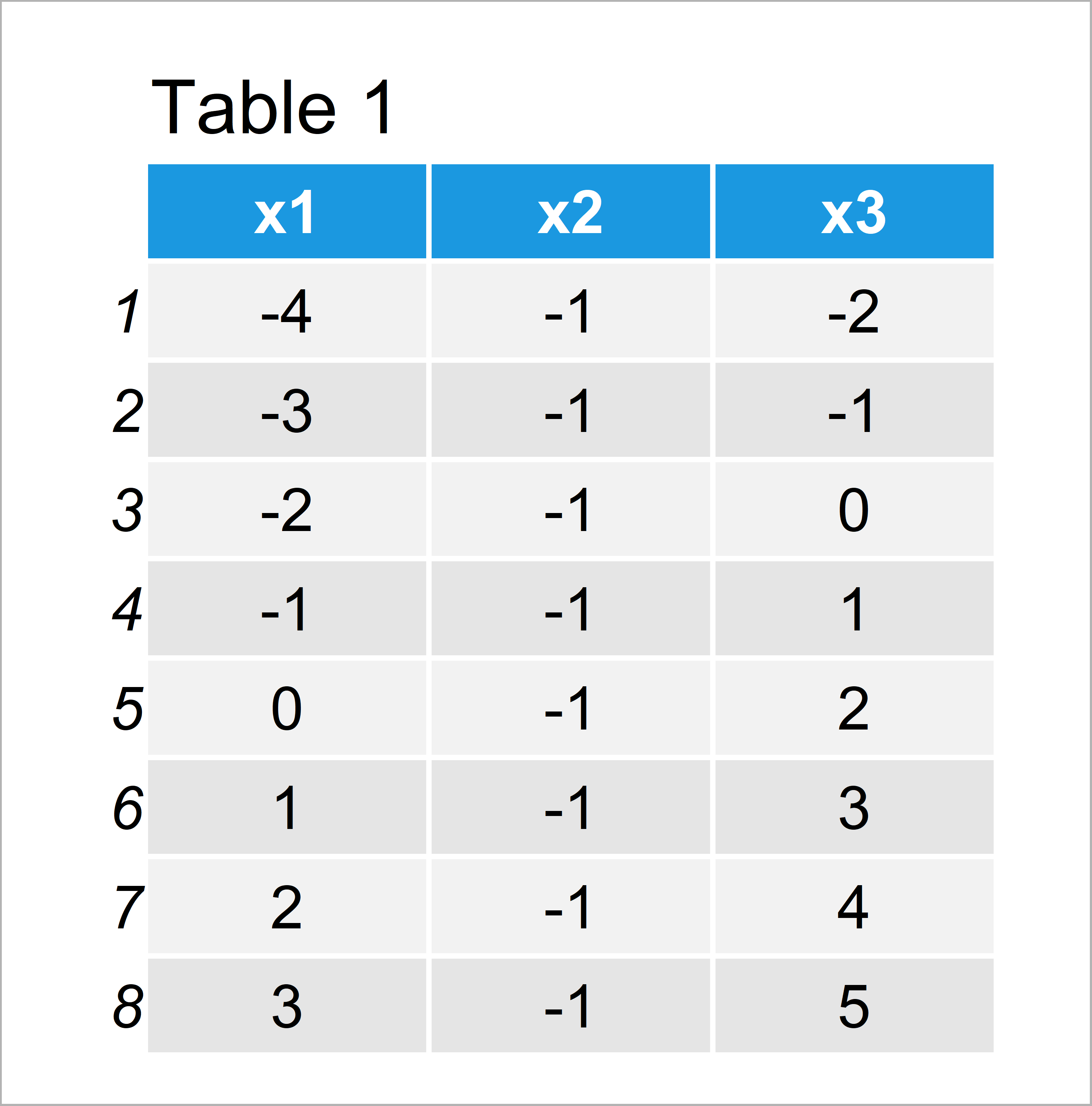# Replace Negative Values by Zero in R (2 Examples)

In this R tutorial you’ll learn how to set negative values to zero.

Here’s how to do it:

## Example 1: Replace Negative Values in Vector by Zero

In this example, I’ll illustrate how to replace negative values with zero in R.

First, we have to create an example vector in R:

```vec <- c(-1, 4, 2, 5, -3, 9, -9, 0, 5)    # Create example vector
vec                                       # Print example vector
#  -1  4  2  5 -3  9 -9  0  5```

The previous output of the RStudio console shows the structure of our vector. It contains different numeric values, whereby some of these values are smaller than zero.

Next, we can exchange all negative numbers by zero using a logical condition:

```vec_positive <- vec                       # Duplicate vector
vec_positive[vec_positive < 0] <- 0       # Set negative values to 0
vec_positive                              # Print updated vector
#  0 4 2 5 0 9 0 0 5```

Have a look at the previous output: We have created a new vector object called vec_positive that contains only positive numbers. In other words, we have converted our vector to a semi-continuous format.

## Example 2: Replace Negative Values in Data Frame by Zero

In Example 2, I’ll explain how to set the negative values in all columns of a data frame to zero.

For this example, we have to construct a data frame first:

```data  <- data.frame(x1 = -4:3,            # Create example data frame
x2 = -1,
x3 = -2:5)
data                                      # Print example data frame```As shown in Table 1, the previously executed syntax has created a data frame with eight rows and three variables. Each of these variables contains some or only negative values.

The following R code replaces all numbers to the value 0 that have a minus sign in front.

```data_positive <- data                     # Duplicate data frame
data_positive[data_positive < 0] <- 0     # Set negative values to 0
data_positive                             # Print updated data frame```In Table 2 it is shown that we have created a new data frame containing only the numbers zero or higher by executing the previous R programming syntax.

## Video & Further Resources

Some time ago I have published a video instruction on my YouTube channel, which shows the R codes of this tutorial. Please find the video below:

Please accept YouTube cookies to play this video. By accepting you will be accessing content from YouTube, a service provided by an external third party.If you accept this notice, your choice will be saved and the page will refresh.

Furthermore, you might read the other articles on this website:

You have learned in this article how to substitute negative numbers by zero in R. Let me know in the comments, in case you have any additional comments or questions.

Subscribe to the Statistics Globe Newsletter